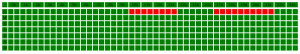# Storing opening and closing times in database for stores

Requirement:
• Store can open and close many times during the day
• Store can close the day after it opens (store opened after midnight)
• Precision of the time must be 1/2 hour / 30minutes
• Searching for the store opened at a certain time must be done efficiently
• Every weeks have same business hours
Info:
• Type "Time" doesn't exists in MySQL
Example:
• Mon : 10.00-14.00 17.00-22.00
• Tue : 10.00-22.00
• Wed : 10.00-22.30
• Thu : 10.00-22.30
• Fri : 10.30-01.00 (+1d)
• Sat : 11.30-02.00 (+1d)
• Sun : Closed

## 1/Encode all the opening-closing time of a store in one number

Let's see the opening time table as an array. For instance if the store would be open from 10am to 2pm and 5pm to 10pm we would have: 000000000000000000001111111100000011111111110000 <=> 17575273562112 20*0 (20 first 1/2 hour closed) ; 8*1 (4 hours / 8 1/2hours openend) ; etc...For one day there are 2^(24*2) = 2^48 possibilities
For one week there are 2^(24*2*7) = 2^336
As the bigger mysql integer size is 2^64 we don't have big enough integer to encode it.
More explication here: http://remy-mellet.com/dev/b48encode.html

## 2/OpeningPeriod table

• id
• store_id
• day [0=sun - 1=mon - ... - 6=sat]
• open [0am=0; 0.30am=1; 1am=2 ... 11pm=46]
• close [0am=0; 0.30am=1; 1am=2 ... 11pm=46 ... 2am(+1d)=52]
We must be able to store opening time during the night, so for the closing time, if it was open the day before we will add 48. If the lastest time is 10am (d+1) <=> 48+20 = 68. So it can be encoded as a Mysql TINYINT ( 2^8 ).
Current time is 1915, Mon => curTime = ceil(19.25*2) = 39 ; curDay = 1 ; prevDay = 0;
Current time is 0130, Sun => curTime = ceil(1.5*2) = 3; curDay = 0; prevDay = 6;
Get the stores id open:
select distinct(p.store_id) from OpeningPeriod p where (day = curDay and open <= curTime and close >= curTime ) OR (day = prevDay and open <= curTime+48 and close >= curTime+48)

## 3/OpeningPeriod table w/o day

To simplify, instead of taking as reference for the opening day the beginning of the day, why not just take the beginning of the week? Thus we will take off the day column.
• id
• store_id
• open [0am/Sun=0; 0.30am/Sun=1; 1am/Sun=2 ... 11pm/Sun=46 ... 6pm/Sat=324 (48*6+18*2)]
• close [0am/Sun=0; 0.30am/Sun=1; 1am/Sun=2 ... 2am/Sun = 4+336 = 340]
The open and close can be encoded as Mysql SMALLINT (2^16)
Current time is 19.15, Mon => curTime = ceil(19.25*2) = 39
Current time is 01.30, Sun => curTime = ceil(1.5*2) = 3
Get the stores id open:
select distinct(p.store_id) from OpeningPeriod p where (open <= curTime and close >= curTime ) OR (open <= curTime and close >= curTime+336)